# Pipelining and vector processing

Self Employed
Jun. 12, 2014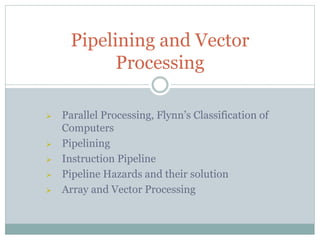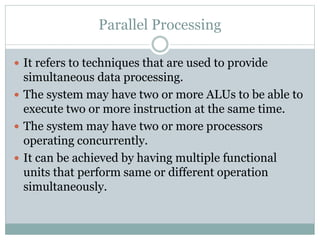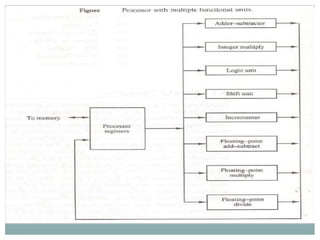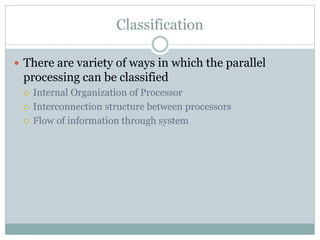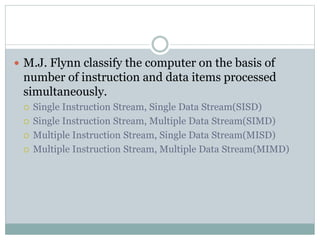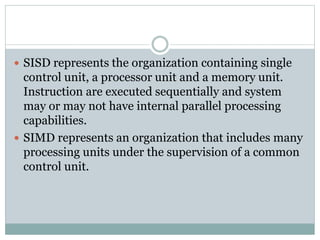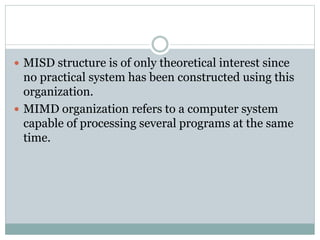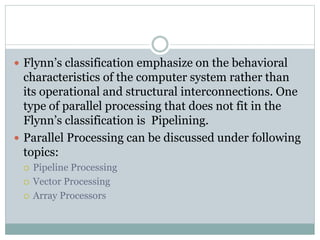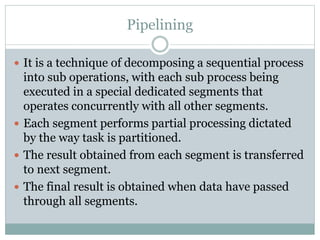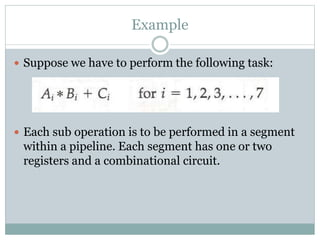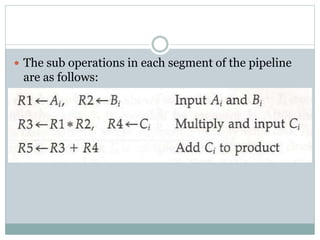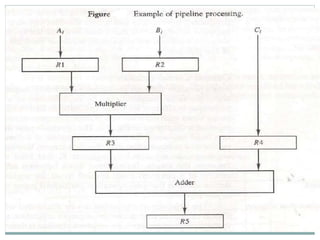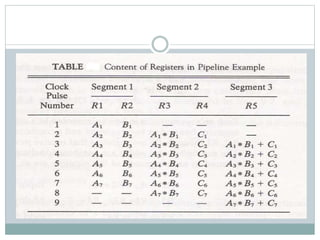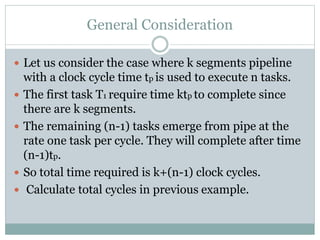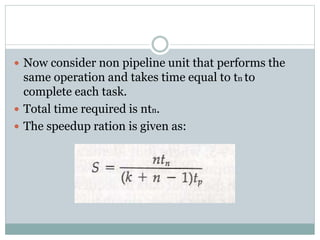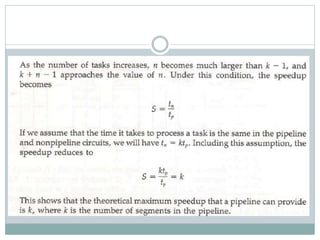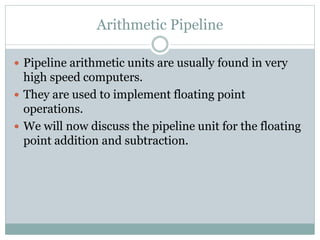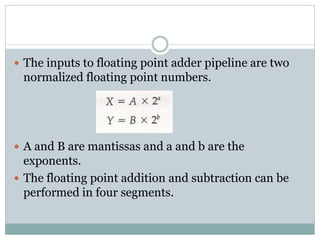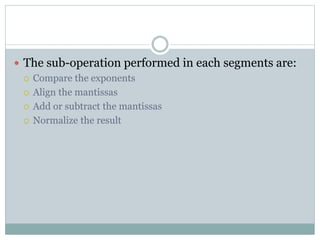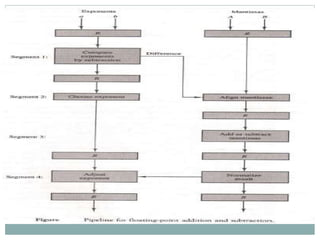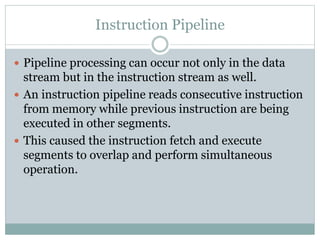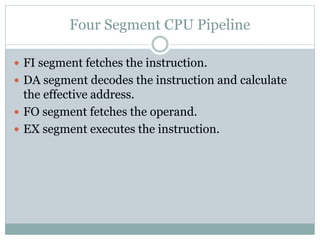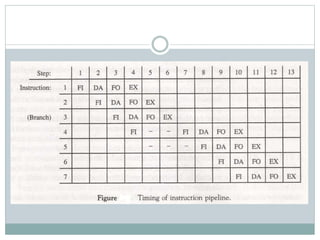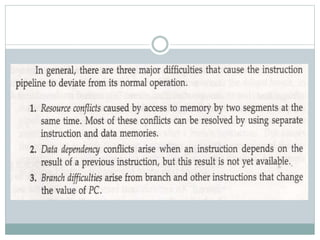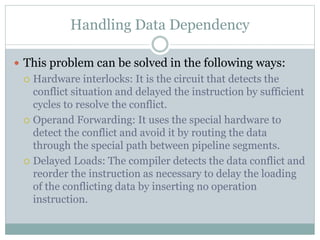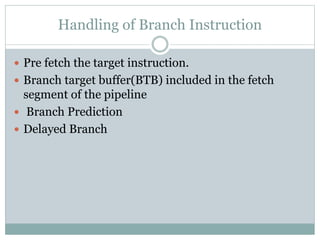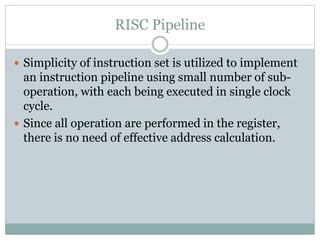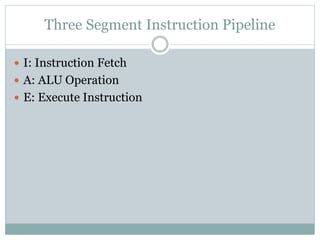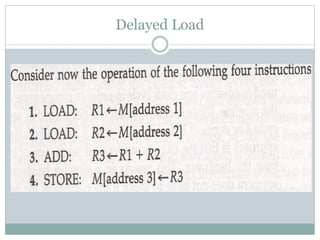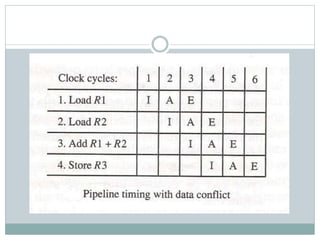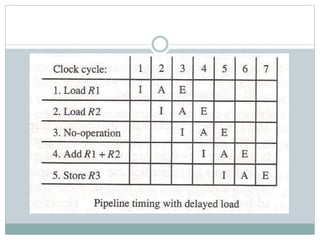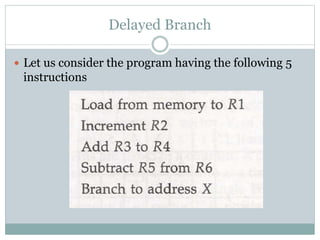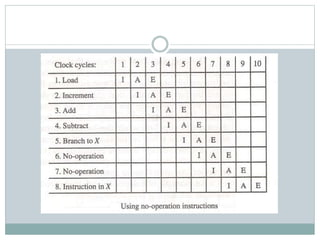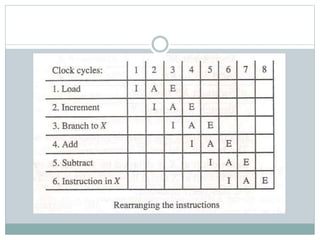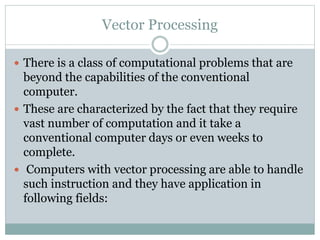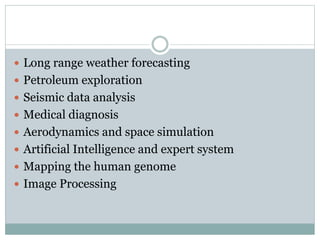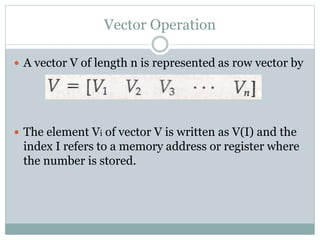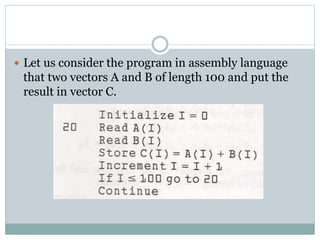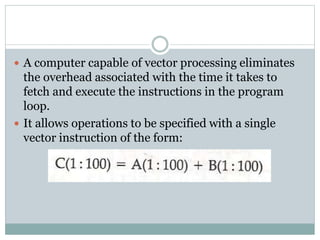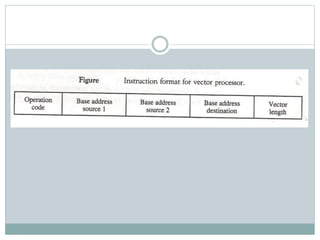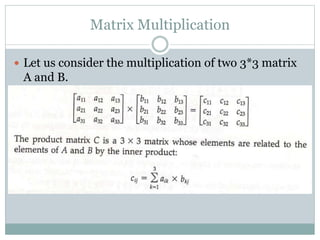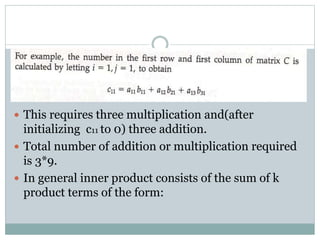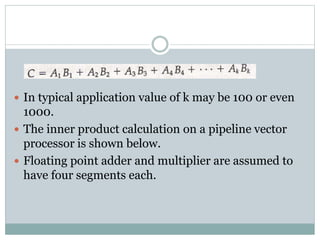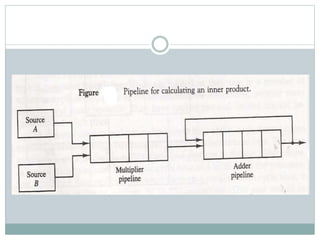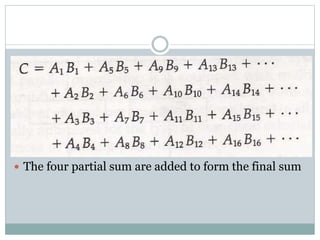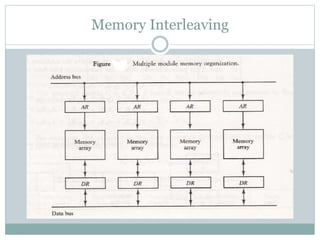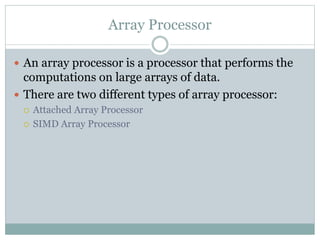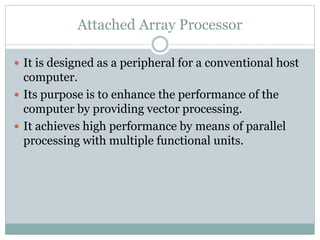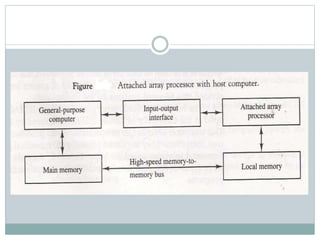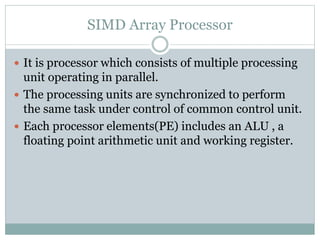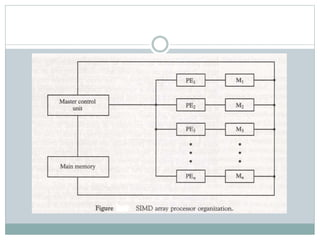1 of 52

### Pipelining and vector processing

• 1.  Parallel Processing, Flynn’s Classification of Computers  Pipelining  Instruction Pipeline  Pipeline Hazards and their solution  Array and Vector Processing Pipelining and Vector Processing
• 2. Parallel Processing  It refers to techniques that are used to provide simultaneous data processing.  The system may have two or more ALUs to be able to execute two or more instruction at the same time.  The system may have two or more processors operating concurrently.  It can be achieved by having multiple functional units that perform same or different operation simultaneously.
• 4. Classification  There are variety of ways in which the parallel processing can be classified  Internal Organization of Processor  Interconnection structure between processors  Flow of information through system
• 5.  M.J. Flynn classify the computer on the basis of number of instruction and data items processed simultaneously.  Single Instruction Stream, Single Data Stream(SISD)  Single Instruction Stream, Multiple Data Stream(SIMD)  Multiple Instruction Stream, Single Data Stream(MISD)  Multiple Instruction Stream, Multiple Data Stream(MIMD)
• 6.  SISD represents the organization containing single control unit, a processor unit and a memory unit. Instruction are executed sequentially and system may or may not have internal parallel processing capabilities.  SIMD represents an organization that includes many processing units under the supervision of a common control unit.
• 7.  MISD structure is of only theoretical interest since no practical system has been constructed using this organization.  MIMD organization refers to a computer system capable of processing several programs at the same time.
• 8.  Flynn’s classification emphasize on the behavioral characteristics of the computer system rather than its operational and structural interconnections. One type of parallel processing that does not fit in the Flynn’s classification is Pipelining.  Parallel Processing can be discussed under following topics:  Pipeline Processing  Vector Processing  Array Processors
• 9. Pipelining  It is a technique of decomposing a sequential process into sub operations, with each sub process being executed in a special dedicated segments that operates concurrently with all other segments.  Each segment performs partial processing dictated by the way task is partitioned.  The result obtained from each segment is transferred to next segment.  The final result is obtained when data have passed through all segments.
• 10. Example  Suppose we have to perform the following task:  Each sub operation is to be performed in a segment within a pipeline. Each segment has one or two registers and a combinational circuit.
• 11.  The sub operations in each segment of the pipeline are as follows:
• 14. General Consideration  Let us consider the case where k segments pipeline with a clock cycle time tp is used to execute n tasks.  The first task T1 require time ktp to complete since there are k segments.  The remaining (n-1) tasks emerge from pipe at the rate one task per cycle. They will complete after time (n-1)tp.  So total time required is k+(n-1) clock cycles.  Calculate total cycles in previous example.
• 15.  Now consider non pipeline unit that performs the same operation and takes time equal to tn to complete each task.  Total time required is ntn.  The speedup ration is given as:
• 17. Arithmetic Pipeline  Pipeline arithmetic units are usually found in very high speed computers.  They are used to implement floating point operations.  We will now discuss the pipeline unit for the floating point addition and subtraction.
• 18.  The inputs to floating point adder pipeline are two normalized floating point numbers.  A and B are mantissas and a and b are the exponents.  The floating point addition and subtraction can be performed in four segments.
• 19.  The sub-operation performed in each segments are:  Compare the exponents  Align the mantissas  Add or subtract the mantissas  Normalize the result
• 21. Instruction Pipeline  Pipeline processing can occur not only in the data stream but in the instruction stream as well.  An instruction pipeline reads consecutive instruction from memory while previous instruction are being executed in other segments.  This caused the instruction fetch and execute segments to overlap and perform simultaneous operation.
• 22. Four Segment CPU Pipeline  FI segment fetches the instruction.  DA segment decodes the instruction and calculate the effective address.  FO segment fetches the operand.  EX segment executes the instruction.
• 26. Handling Data Dependency  This problem can be solved in the following ways:  Hardware interlocks: It is the circuit that detects the conflict situation and delayed the instruction by sufficient cycles to resolve the conflict.  Operand Forwarding: It uses the special hardware to detect the conflict and avoid it by routing the data through the special path between pipeline segments.  Delayed Loads: The compiler detects the data conflict and reorder the instruction as necessary to delay the loading of the conflicting data by inserting no operation instruction.
• 27. Handling of Branch Instruction  Pre fetch the target instruction.  Branch target buffer(BTB) included in the fetch segment of the pipeline  Branch Prediction  Delayed Branch
• 28. RISC Pipeline  Simplicity of instruction set is utilized to implement an instruction pipeline using small number of sub- operation, with each being executed in single clock cycle.  Since all operation are performed in the register, there is no need of effective address calculation.
• 29. Three Segment Instruction Pipeline  I: Instruction Fetch  A: ALU Operation  E: Execute Instruction
• 33. Delayed Branch  Let us consider the program having the following 5 instructions
• 36. Vector Processing  There is a class of computational problems that are beyond the capabilities of the conventional computer.  These are characterized by the fact that they require vast number of computation and it take a conventional computer days or even weeks to complete.  Computers with vector processing are able to handle such instruction and they have application in following fields:
• 37.  Long range weather forecasting  Petroleum exploration  Seismic data analysis  Medical diagnosis  Aerodynamics and space simulation  Artificial Intelligence and expert system  Mapping the human genome  Image Processing
• 38. Vector Operation  A vector V of length n is represented as row vector by  The element Vi of vector V is written as V(I) and the index I refers to a memory address or register where the number is stored.
• 39.  Let us consider the program in assembly language that two vectors A and B of length 100 and put the result in vector C.
• 40.  A computer capable of vector processing eliminates the overhead associated with the time it takes to fetch and execute the instructions in the program loop.  It allows operations to be specified with a single vector instruction of the form:
• 42. Matrix Multiplication  Let us consider the multiplication of two 3*3 matrix A and B.
• 43.  This requires three multiplication and(after initializing c11 to 0) three addition.  Total number of addition or multiplication required is 3*9.  In general inner product consists of the sum of k product terms of the form:
• 44.  In typical application value of k may be 100 or even 1000.  The inner product calculation on a pipeline vector processor is shown below.  Floating point adder and multiplier are assumed to have four segments each.
• 46.  The four partial sum are added to form the final sum
• 48. Array Processor  An array processor is a processor that performs the computations on large arrays of data.  There are two different types of array processor:  Attached Array Processor  SIMD Array Processor
• 49. Attached Array Processor  It is designed as a peripheral for a conventional host computer.  Its purpose is to enhance the performance of the computer by providing vector processing.  It achieves high performance by means of parallel processing with multiple functional units.
• 51. SIMD Array Processor  It is processor which consists of multiple processing unit operating in parallel.  The processing units are synchronized to perform the same task under control of common control unit.  Each processor elements(PE) includes an ALU , a floating point arithmetic unit and working register.